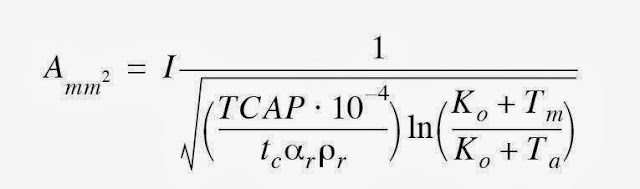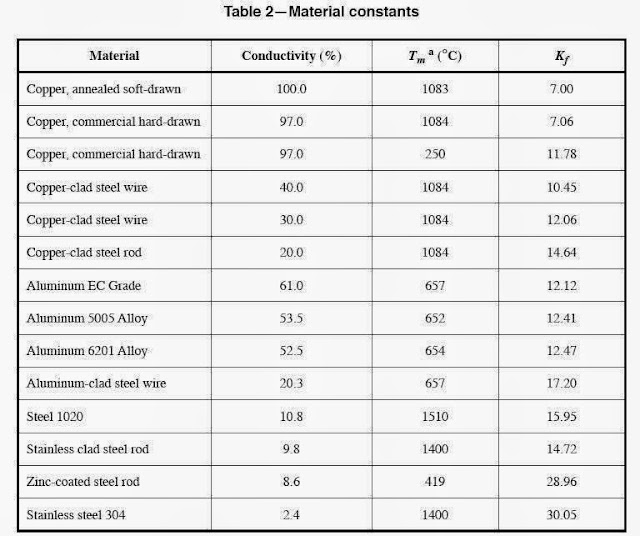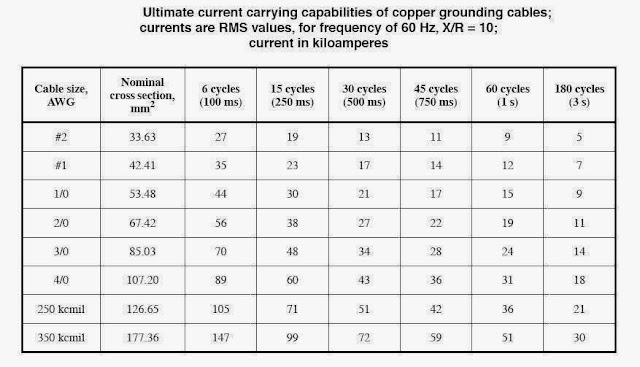Grounding Design Calculations – Part Ten

In Article
Grounding Design Calculations – Part One ", I indicated the following:

 Grounding System Design Calculations according to type of the building The procedures for performing the Grounding System Design Calculations can differ slightly according to the type of the building as follows: Domestic, commercial and industrial premises, High and medium voltage electricity substations.

 First: Domestic, commercial and industrial premises We mean by domestic, commercial and industrial premises, all installations up to 1,000 V ac and 1,500 V dc - between phases, with some minor exceptions.

And I explained Methods of Grounding Design Calculations of Domestic, commercial and industrial premises in the following Articles:

Today, I will explain Grounding Design Calculations of High and Medium Voltage Electricity AC Substations.

 Grounding System Design Calculations Of High And Medium Voltage AC Substations

 1- Standards Standard used for ground calculations of High and medium voltage electricity AC substations is IEEE 80: Guide for safety in AC substation grounding. Note: This standard is primarily concerned with outdoor ac substations, either conventional or gas-insulated. Distribution, transmission, and generating plant substations are included. With proper caution, the methods described herein are also applicable to indoor portions of such substations, or to substations that are wholly indoors. It doesn’t cover the grounding problems peculiar to dc substations.

2- Design Procedures

The design process of a substation grounding system requires many steps. The following steps were established by the IEEE Standard 80-2000 for the design of the ground grid:

• Step#1: Field Data Collection,
• Step#2: Earthing Grid Conductor Sizing,
• Step#3: Calculation of tolerable touch and step voltages,
• Step#4: Preliminary design of grounding system,
• Step#5: Calculation of of the preliminary Grid Resistance, RG, of the grounding system in uniform soil.
• Step#6: Determination of Grid current, IG.
• Step#7: Calculation of maximum grid potential rise and comparing with the tolerable touch voltage from step#3. If the GPR of the preliminary design is below the tolerable touch voltage, move to step#12 (no further analysis is necessary). If not, continue to step#8.
• Step#8: Calculation of mesh and step voltages.
• Step#9: Comparing the computed mesh voltage from step#8 with the tolerable touch voltage from step#3. If the computed mesh voltage is below the tolerable touch voltage, continue to step#10. If not, move to step#11 for revising the preliminary design.
• Step#10: Comparing the computed step voltage from step#8 with the tolerable step voltage from step#3.If the computed step voltages are below the tolerable step voltage, move to step#12. If not, move to step#11 for revising the preliminary design.
• Step#11: Preliminary Design modification, If either the step or touch tolerable limits from step#3 are exceeded, revision of the grid design is required.
• Step#12: Detailed final design. After satisfying the step and touch voltage requirements, additional grid and ground rods /conductors may be required. The final design should also be reviewed to eliminate hazards due to transferred potential and hazards associated with special areas of concern.

The block diagram in Fig (1) illustrates the Design procedures.Fig (1)

In this Article and following Articles, I will explain these steps in detail.

 Step#1: Field Data Collection The following information is required / desirable before starting the calculation: A layout of the site, Maximum earth fault current into the earthing grid, Maximum fault clearing time, Back up time, Ambient (or soil) temperature at the site, Soil resistivity measurements at the site (for touch and step only), Resistivity of any surface layers intended to be laid (for touch and step only), Location of the feeding station, Near-by utility, Communication network in the area, Feeding characteristics, Any special considerations.

 Step#2: Earthing Grid Conductor Sizing In this step, we will know how to size the earthing grid conductor in the following two cases: Symmetrical Fault currents (with no dc offset), Asymmetrical Fault currents.

Terms Definitions for Step#2

• Grounding System: it is the system that Comprises all interconnected grounding facilities in a specific area.
• dc Offset: Difference between the symmetrical current wave and the actual current wave during a power system transient condition, see Fig (2). Mathematically, the actual fault current can be broken into two parts, a symmetrical alternating component and a unidirectional (dc) component. The unidirectional component can be of either polarity, but will not change polarity, and will decrease at some predetermined rate.Fig (2)

• Decrement Factor: An adjustment factor used in conjunction with the symmetrical ground fault current parameter in safety-oriented grounding calculations. It determines the rms equivalent of the asymmetrical current wave for a given fault duration, tf, accounting for the effect of initial dc offset and its attenuation during the fault.
• IF Effective Asymmetrical Fault Current: The rms value of asymmetrical current wave, integrated over the interval of fault duration.
• Grounding Grid: A system of horizontal ground electrodes that consists of a number of interconnected, bare conductors buried in the earth, providing a common ground for electrical devices or metallic structures, usually in one specific location.

Note:

1. Grids buried horizontally near the earth’s surface are also effective in controlling the surface potential gradients.
2. A typical grid usually is supplemented by a number of ground rods and may be further connected to auxiliary ground electrodes to lower its resistance with respect to remote earth.

 First: Earthing Grid Conductor Sizing For Symmetrical Fault Currents

Earthing Grid Conductor Sizing for Symmetrical Fault Currents

The minimum conductor size of the earthing grid conductors capable of withstanding the adiabatic temperature rise associated with an earth fault is given by re-arranging IEEE Std 80 Equation 37 (which is taken from the derivation by Sverak):Where:

I is the rms current in kA
Amm2 is the conductor cross section in mm2
Tm is the maximum allowable temperature in °C
Ta is the ambient temperature in °C
Tr is the reference temperature for material constants in °C
αo is the thermal coefficient of resistivity at 0 °C in 1/°C
αr is the thermal coefficient of resistivity at reference temperature Tr in 1/°C
ρr is the resistivity of the ground conductor at reference temperature Tr in μΩ-cm
Ko = [(1/αo– Tr  )]or [(1/αr) – Tr]  in °C
tc is the duration of current in s
TCAP is the thermal capacity per unit volume from Table-1, in J/(cm3·°C)

Notes:

1- It should be noted that αr and ρr are both to be found at the same reference temperature of Tr °C. Table-1 provides data for αr and ρr at 20 °C.Table-1

2- If the conductor size is given in kcmils:

Since, kcmils = mm2 × 1.974 , so the above Equation becomes as follows:

3- calculation of TACP for materials not listed in Table-1:

TCAP can be calculated for materials not listed in Table-1 from the specific heat and specific weight. Specific heat, SH, in cal/(grams × °C) and specific weight, SW, in gram/cm3 are related to the thermal capacity per unit volume in J/(cm3 × °C) as follows:

Since 1 calorie  =  4.184 J

Therefore, TCAP is defined by;

TCAP [cal/(cm3 · _°C)] = SH [cal/(gram · °C)] · SW (gram/cm3)
or
TCAP [J/(cm3 ·°C)] = 4.184 (J/cal) · SH [(cal/(gram · °C)] · SW (gram/cm3)

4- The Equation in English units can be simplified to the following:

Akcmil = I x Kf x √ tc

Where:

Akcmil is the area of conductor in kcmil,
I is the rms fault current in kA,
tc is the current duration in s,
Kf is the constant from Table-2 for the material at various values of
Tm (fusing temperature or limited conductor temperature) and using ambient temperature (Ta) of 40°C.Table-2

 Example#1: Calculate the Earthing Grid Conductor Size for 30% copper-clad steel conductor for 1 KA fault current and duration = 1 sec. Solution: For instance, to calculate the 1 s size of a 30% copper-clad steel conductor, one gets tc = 1.0, α20 = 0.003 78, ρ20 = 5.86, TCAP = 3.85, Tm = 1084, Ta = 40, K0 = 245 Thus, for I = 1 kA Akcmil = 197.4/ √267.61 = 12.06 k cmil or 12.06 kcmil/kA Example#2: A 20 kA, 3 s fault current, calculate the Earthing Grid Conductor Size using, the English unit equation, for each of the following conductor’s material: Soft drawn copper conductor, 40% conductivity copper-clad steel conductor, Steel conductor. Solution: 1- For soft drawn copper Akcmil = 20 x7.00√3  = 242.5 kcmil use 250 kcmil 2- For 40% conductivity copper-clad steel conductor Akcmil = 20x 10.45 = 362.0 kcmil use 19/#7 conductor 3- For steel conductor Akcmil = 20 x 15.95 = 552.5 kcmil use 7/8 inch diameter conductor

 Second: Earthing Grid Conductor Sizing For Asymmetrical Fault Currents

Earthing Grid Conductor Sizing For Asymmetrical Fault Currents

The minimum conductor size of the earthing grid conductors in case of Asymmetrical Fault Currents can be calculated by one of the following two methods:

Method#1: Using Decrement Factor

In cases where accounting for a possible dc offset component in the fault current is desired, an equivalent value of the symmetrical current, IF, will be used in above equations for Earthing Grid Conductor Sizing For Symmetrical Fault Currents as follows:

IF=  If x Df
Where:

If  is the symmetrical fault current,
Df is the Decrement Factor.

Notes:

1. The resulting value of IF is always larger than If,
2. The effect of dc offsets can be neglected if the duration of the current is greater than or equal to 1 s or the X/R ratio at the fault location is less than 5.

Calculation of Decrement Factor Df:

The following Equation can be used to compute the decrement factor for specific X/R ratios and fault durations:

Where:

Ta is the dc offset time constant in s [Ta = X/(ωR), for 60 Hz, Ta = X/(120πR)],
tf is the time duration of fault in s.

However, Typical values of the decrement factor for various fault durations and X/R ratios are shown in Table-3.Table-3

Method#2: Using Asymmetrical Current Tables

Fusing characteristics for various sizes of copper conductor with various degree of dc offset are presented in Table-4 through Table-7 as follows:Table-4Table-5Table-6Table-7

Notes:

1. The current values in Table 3 through Table 6 were computed from the computer program RTGC (Reichman, Vainberg, and Kuffel). This computer program can be used directly to determine the grounding cable size requirements for known X/R ratio and fault clearing time.
2. Current is computed for maximum dc offset.
3. Initial conductor temperature = 40 °C; final conductor temperature = 1083 °C.
4. Metric values are soft conversions. Soft conversion is a direct area calculation, in metric units, from the AWG size.

 Additional Conductor Sizing Factors It may be prudent to choose a larger conductor size to compensate for some gradual reduction in the conductor cross-section because of corrosion during the design life of the installation where the soil environment tends to promote corrosion. The down leads may have to be larger than the grid conductors or may have to be multiples from the equipment to the grid to have sufficient ampacity for the total fault current. Ground lead conductors conducting lightning current, the size of these conductors must be also adequate for carrying short time surges caused by lightning. Considering The possibility of future growth. It is less costly to include an adequate margin in conductor size during the initial design than to try to reinforce a number of ground leads at a later date.

In the next Article, I will explain Other Steps from the Design Procedures of Grounding System Design for AC Substation. Please, keep following.

1 comment:

1.Very good site having too much information